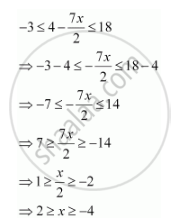CBSE (Arts) Class 11CBSE
Share

# Solve the Inequality -3 <= 4 - (7x)/2 < = 18 - CBSE (Arts) Class 11 - Mathematics

ConceptAlgebraic Solutions of Linear Inequalities in One Variable and Their Graphical Representation

#### Question

Solve the inequality -3 <= 4 - (7x)/2  < = 18

#### SolutionThus, the solution set for the given inequalityis [–4, 2].

Is there an error in this question or solution?

#### APPEARS IN

Solution Solve the Inequality -3 <= 4 - (7x)/2 < = 18 Concept: Algebraic Solutions of Linear Inequalities in One Variable and Their Graphical Representation.
S